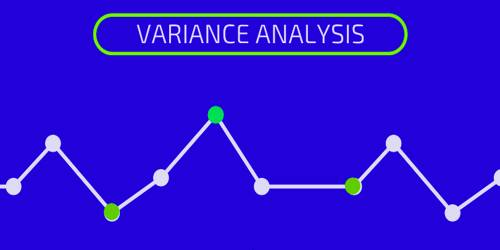# Variance Analysis

Variance analysis is a tool of budgetary control by evaluation of performance by means of variances between budgeted amount, planned amount or standard amount and the actual amount incurred/sold. It is the quantitative investigation of the difference between actual and planned behavior. Variance analysis can be carried out for both costs and, revenues. It is generally related to a manufacturer’s product costs.

Variance analysis is usually associated with explaining the difference between actual costs and the standard costs allowed for the good output. It is the study of deviations of actual behavior versus forecasted or designed behavior in budgeting or management accounting. For example, the difference in materials costs can be divided into a materials Price variance and a materials usage variance. The difference between the actual direct labor costs and the standard direct labor costs can be divided into a rate variance and an efficiency variance. The difference in manufacturing overhead can be divided into spending, efficiency, mid-volume variances. Mix and yield variances can also be calculated.

Variance analysis helps management to understand the present costs and then to control future costs. It is a methodical tool that managers can use to evaluate actual operations to budgeted estimates.